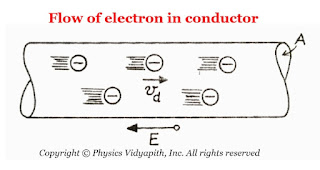### Relation between electric current and drift velocity

Derivation→ Let us consider

• The length of the conductor = $l$

• The cross-section area of the conductor = $A$

• The total number of free electrons inside the conductor = $N$

• The current flow in the conductor = $i$

•The flow of Electron in Conductor
• The Relaxation time between the two successive collisions =$\tau$

• According to the law of current density

$J=\frac{i} {A}$

$J=\frac{q} {A \tau} \qquad \left( \because i=\frac{q}{\tau} \right)$

$J=\frac{N \: e }{A \tau} \qquad \left( \because q=Ne \right)$

Now multiply by length of conductor $l$ in above equation. Therefore we get

$J=\frac{N \: e \: l}{A \tau\: l}$

$J=\frac{N \: e \: l}{V \tau\: }\qquad \left(\because V=A.l \right)$

Where $V$ is volume of the conductor.

$J=\frac{n \: e \: l}{ \tau }\qquad \left( \because n=\frac{N}{V} \right)$

Where $n$ is total number of electrons per unit .

$J=n \: e \: v_{d}\qquad \left( \because v_{d}=\frac{l}{\tau}\right)$

Where $v_{d}$ are known as drift velocity of charged particles.

Now substitute the value of current density $J$ from equation $(1)$ to above equation then above equation can be written as

 $i=neAv_{d}$